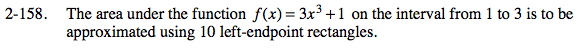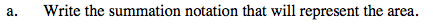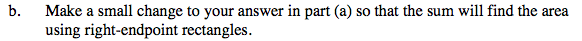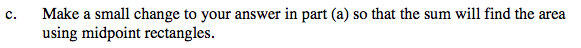### Home > PC > Chapter 2 > Lesson 2.3.7 > Problem2-158

2-158.Sketch the function.

Draw 10 rectangles between x = 1 and 3.

What is the width for each rectangle? What are the x-values for the heights?

What expression needs to go into f(x) to convert the integer values to 1, 1.2, 1.4, 1.6..., 2.8?

Write the summation now. Be careful with your k-values.

$\frac{3-1}{10}=\frac{2}{10}=0.2$

x0 = 1; x1 = 1.2; x2 = 1.4; x3 = 1.6; ...; x9 = 2.8

0.2k + 1What k-values should you start and stop with?Change the inside function to: 0.2k + 1.1 so that it is starting at the middle of each rectangle.